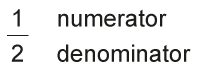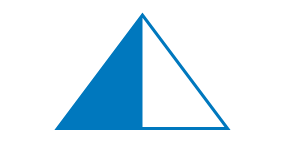Home > Fractions > Big ideas > Part-whole fractions

# Part-whole fractions

In the part-whole interpretation, the denominator shows the number of equal parts in the whole and the numerator shows how many of those parts are included in the particular fraction.

The part-whole construct is often represented with an area model, such as a shape partitioned into equal parts.

For example, the area of this triangle has been partitioned into two equal areas. The fraction $$\frac 1 2$$ would refer to 'one part out of two equal parts' of the area of the triangle.An area model showing halves.

The part-whole use of fractions applies in other practical contexts involving physical quantities, such as length, volume and groups of objects. It can also be applied to more abstract contexts such as time, as in 'a quarter of an hour' or 'half past five'.

## Using the part-whole model

The part-whole model offers a helpful introduction to fractions. It is also useful for exploring equivalent fractions and the addition and subtraction of fractions, but there are some potential pitfalls.